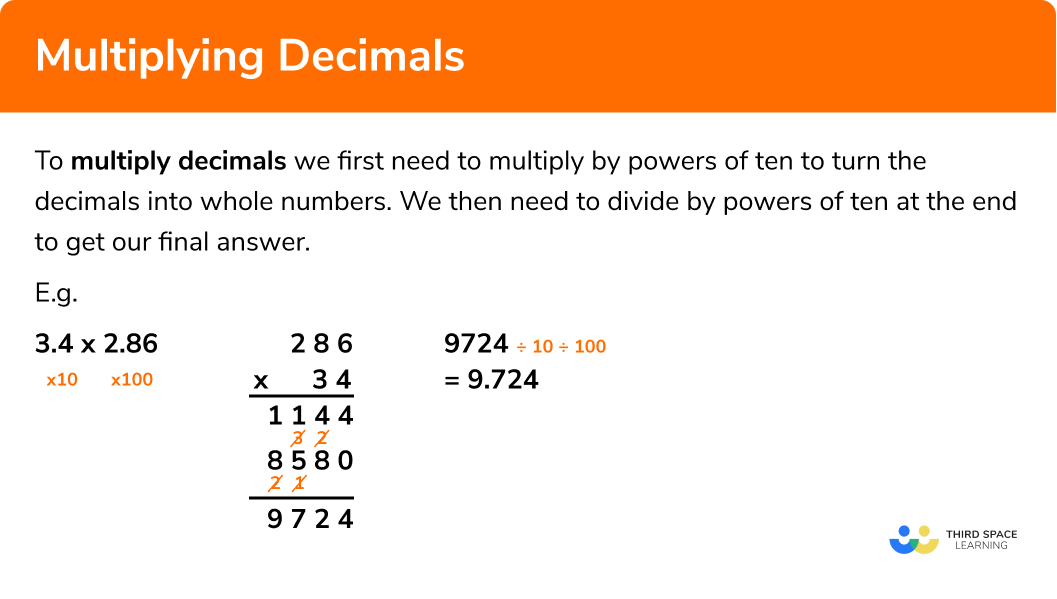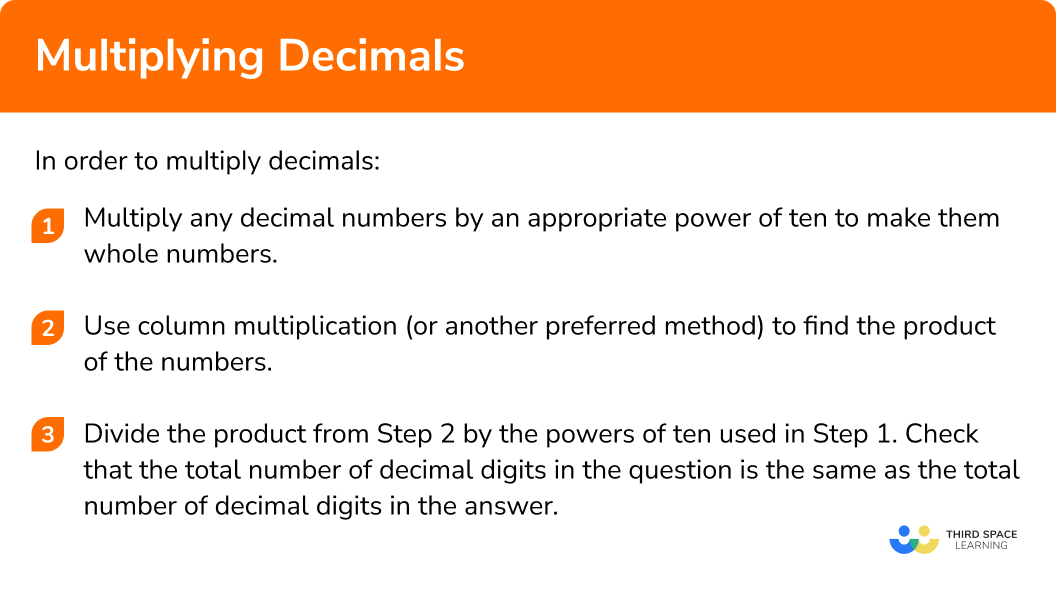# Multiplying Decimals

Here we will learn about multiplying decimals including how to multiply decimals by a whole number and multiply decimals by decimals.

There are also multiplying decimals worksheets and word problems based on Edexcel, AQA and OCR exam questions, along with further guidance on where to go next if you’re still stuck.

## What is multiplying decimals?

Multiplying decimals is where we multiply together decimal numbers.
To multiply decimals we first need to multiply by powers of ten to turn the decimals into whole numbers. We then need to divide by powers of ten at the end to get our final answer.

Multiplying decimals is important when dealing with money problems such as the conversion of currency. It is also a vital skill when converting between metric units and other multiplication problems.

E.g.

### What is multiplying decimals?### Recap of multiplying and dividing by 10, 100, 1000…

When solving problems where we need to multiply or divide decimals, we need to multiply and divide by powers of ten.
When we multiply by a power of ten, each digit increases in place value.

If we multiply by 10, the digits move one place to the left, so for example a digit in the hundredths column will move to the tenths column.

E.g.

If we divide by 100, the digits move two places to the right so for example a digit in the tens column will move to the tenths column.

E.g.

## How to multiply decimals

To multiply decimals we will multiply by powers of ten at the start, and then divide by powers of ten at the end to get our final answer.

In order to multiply decimals:

1. Multiply any decimal numbers by an appropriate power of ten to make them whole numbers.
2. Use column multiplication (or another preferred method) to find the product of the numbers.
3. Divide the product from Step 2 by the powers of ten used in Step 1. Check that the total number of decimal digits in the question is the same as the total number of decimal digits in the answer.

### Explain how to multiply decimals in 3 steps### Related lessons on decimals

Multiplying decimals is part of our series of lessons to support revision on decimals. You may find it helpful to start with the main decimals lesson for a summary of what to expect, or use the step by step guides below for further detail on individual topics. Other lessons in this series include:

## Multiplying decimals examples

### Example 1: multiplying an integer by a decimal

Work out

6 × 5.2

1. Multiply any decimal numbers by an appropriate power of ten to make them whole numbers.

Multiply 5.2 by 10 to make it 52.

2Use column multiplication (or another preferred method) to find the product of the numbers.

6 × 52 = 312

3Divide the product from Step 2 by the powers of ten used in Step 1. Check that the total number of decimal digits in the question is the same as the total number of decimal digits in the answer.

In Step 1 we multiplied 5.2 by 10, so now divide by 10.

312 \div 10 = 31.2

Check that the total number of decimal places in the question matches the total number of decimal places in the answer.

### Example 2: multiplying an integer by a decimal

Work out

14 × 3.65

Multiply 3.65 by 100 to make it 365.

14 × 365 = 5110

In Step 1 we multiplied 3.65 by 100, so now divide by 100.

5110 \div 100 = 51.10

Check that the total number of decimal places in the question matches the total number of decimal places in the answer.

The trailing zero can now be removed.

### Example 3: multiplying a decimal by a decimal

Work out

3.7 × 4.9

Multiply 3.7 by 10 to make it 37 and multiply 4.9 by 10 to make it 49.

37 × 49 = 1813

In Step 1 we multiplied 3.7 by 10 and 4.9 by 10.
10 × 10 = 100 so now divide by 100.

1813 \div 100 = 18.13

Check that the total number of decimal places in the question matches the total number of decimal places in the answer.

### Example 4: multiplying a decimal by a decimal

Work out

2.87 × 6.3

Multiply 2.87 by 100 to make it 287 and multiply 6.3 by 10 to make it 63.

287 × 63 = 18081

In Step 1 we multiplied 2.87 by 100 and 6.3 by 10.
100 × 10 = 1000 so now divide by 1000.

18081 \div 1000 = 18.081

Check that the total number of decimals in the question matches the total number of decimals in the answer.

### Common misconceptions

• Decimal point in the wrong position in the answer

It is very common for the answer to have the decimal point in the wrong position. It is important to remember the powers of ten that were used at the start in order to reverse them to calculate the final answer.
Checking that the total number of decimal places in the question is the same as the total number of decimal places in the answer is a way to avoid having the decimal point in the wrong position.
Also rounding the numbers in the question to 1 significant figure and estimating the answer can give a good idea of the size of the actual answer.

E.g.

### Practice multiplying decimals questions

1. Multiply together 6 and 2.8

1.6816.812.48168Work out 6\times28 and then divide the answer by 10

2. Work out 9.4\times16

150.41504144.6415.04Work out 94\times16 and then divide the answer by 10

3. Work out 23\times0.17

39139.123.1613.91Work out 23\times17 and then divide the answer by 100

4. Multiply together 5.6 and 9.8

548854.8845.4815.4Work out 56\times98 and then divide the answer by 100

5. Find the product of 4.83 and 7.2

347.7628.16634.77629.66Work out 483\times72 and then divide the answer by 1000

6. Work out 2\times3.7\times1.54

11.396113966.3787.24Work out 2\times37\times154 and then divide the answer by 1000

### Multiplying decimals GCSE questions

1. You are given that 132\times451 = 59532 . Use this information to work out the following:

(a) 13.2\times451

(b) 1.32\times4.51

(c) 13.2\times0.451

(3 marks)

(a) 5953.2

(1)

(b) 5.9532

(1)

(c) 5.9532

(1)

2. (a) Find the cost of 12 bottles of sparkling water costing £1.69 each.

(b) At a cinema, adult tickets cost £7.75 and child tickets cost £5.49 . Find the total cost of 5 adults and 7 children

(5 marks)

(a) 12 \times 169

(1)

£20.28 i

(1)

(b) 5 \times7.75 or 7\times5.49

(1)

38.75 or 38.43

(1)

(1)

3. Calculate the area of the rectangle(2 marks)

12.4\times8.7 i

(1)

107.88 i

(1)

## Learning checklist

You have now learned how to:

• Understand and use place value for decimals, measures and integers of any size
• Use the four operations, including formal written methods, applied to integers, decimals, proper and improper fractions, and mixed numbers, all both positive and negative

## Still stuck?

Prepare your KS4 students for maths GCSEs success with Third Space Learning. Weekly online one to one GCSE maths revision lessons delivered by expert maths tutors.

Find out more about our GCSE maths tuition programme.